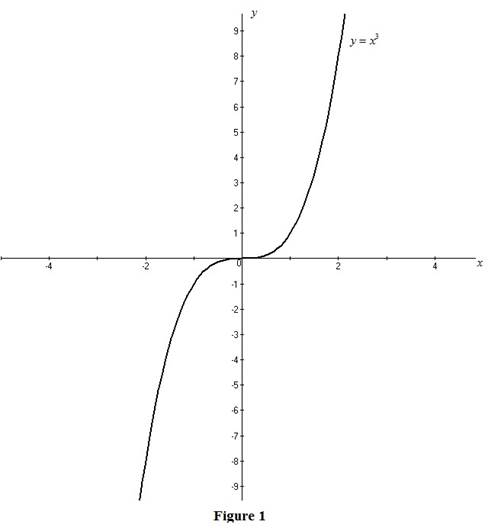# The graph of the function y = ( x − 2 ) 3 by using transformation.### Single Variable Calculus: Concepts...

4th Edition
James Stewart
Publisher: Cengage Learning
ISBN: 9781337687805### Single Variable Calculus: Concepts...

4th Edition
James Stewart
Publisher: Cengage Learning
ISBN: 9781337687805

#### Solutions

Chapter 1, Problem 11RE
To determine

## To sketch: The graph of the function y=(x−2)3 by using transformation.

Expert Solution

### Explanation of Solution

Solution:

The standard graph of the function y=x3 is roughly drawn as shown below in Figure 1.Then, to draw the graph of y=(x2)3, shift the graph y=x3  two units to the right side. Thus, the graph of y=(x2)3 is shown below in Figure 2.Observe that Figure 2 is obtained from Figure 1 in such a way that it is shifted 2 units right side.

### Have a homework question?

Subscribe to bartleby learn! Ask subject matter experts 30 homework questions each month. Plus, you’ll have access to millions of step-by-step textbook answers!